# Deep Q-Learning (DQN)

## Overview

As an extension of the Q-learning, DQN's main technical contribution is the use of replay buffer and target network, both of which would help improve the stability of the algorithm.

Original papers:

## Implemented Variants

Variants Implemented Description
dqn_atari.py, docs For playing Atari games. It uses convolutional layers and common atari-based pre-processing techniques.
dqn.py, docs For classic control tasks like CartPole-v1.
dqn_atari_jax.py, docs For playing Atari games. It uses convolutional layers and common atari-based pre-processing techniques.
dqn_jax.py, docs For classic control tasks like CartPole-v1.

Below are our single-file implementations of DQN:

## dqn_atari.py

The dqn_atari.py has the following features:

• For playing Atari games. It uses convolutional layers and common atari-based pre-processing techniques.
• Works with the Atari's pixel Box observation space of shape (210, 160, 3)
• Works with the Discrete action space

### Usage

poetry install -E atari
python cleanrl/dqn_atari.py --env-id BreakoutNoFrameskip-v4
python cleanrl/dqn_atari.py --env-id PongNoFrameskip-v4

poetry install -E atari
poetry run python cleanrl/dqn_atari.py --env-id BreakoutNoFrameskip-v4
poetry run python cleanrl/dqn_atari.py --env-id PongNoFrameskip-v4

pip install -r requirements/requirements-atari.txt
python cleanrl/dqn_atari.py --env-id BreakoutNoFrameskip-v4
python cleanrl/dqn_atari.py --env-id PongNoFrameskip-v4


### Explanation of the logged metrics

Running python cleanrl/dqn_atari.py will automatically record various metrics such as actor or value losses in Tensorboard. Below is the documentation for these metrics:

• charts/episodic_return: episodic return of the game
• charts/SPS: number of steps per second
• losses/td_loss: the mean squared error (MSE) between the Q values at timestep $$t$$ and the Bellman update target estimated using the reward $$r_t$$ and the Q values at timestep $$t+1$$, thus minimizing the one-step temporal difference. Formally, it can be expressed by the equation below. $$J(\theta^{Q}) = \mathbb{E}_{(s,a,r,s') \sim \mathcal{D}} \big[ (Q(s, a) - y)^2 \big],$$ with the Bellman update target is $$y = r + \gamma \, Q^{'}(s', a')$$ and the replay buffer is $$\mathcal{D}$$.
• losses/q_values: implemented as qf1(data.observations, data.actions).view(-1), it is the average Q values of the sampled data in the replay buffer; useful when gauging if under or over estimation happens.

### Implementation details

dqn_atari.py is based on (Mnih et al., 2015)1 but presents a few implementation differences:

1. dqn_atari.py use slightly different hyperparameters. Specifically,
• dqn_atari.py uses the more popular Adam Optimizer with the --learning-rate=1e-4 as follows:
optim.Adam(q_network.parameters(), lr=1e-4)

whereas (Mnih et al., 2015)1 (Exntended Data Table 1) uses the RMSProp optimizer with --learning-rate=2.5e-4, gradient momentum 0.95, squared gradient momentum 0.95, and min squared gradient 0.01 as follows:
optim.RMSprop(
q_network.parameters(),
lr=2.5e-4,
momentum=0.95,
# ... PyTorch's RMSprop does not directly support
# so we are not sure what to put here.
)

• dqn_atari.py uses --learning-starts=80000 whereas (Mnih et al., 2015)1 (Exntended Data Table 1) uses --learning-starts=50000.
• dqn_atari.py uses --target-network-frequency=1000 whereas (Mnih et al., 2015)1 (Exntended Data Table 1) uses --target-network-frequency=10000.
• dqn_atari.py uses --total-timesteps=10000000 (i.e., 10M timesteps = 40M frames because of frame-skipping) whereas (Mnih et al., 2015)1 uses --total-timesteps=50000000 (i.e., 50M timesteps = 200M frames) (See "Training details" under "METHODS" on page 6 and the related source code run_gpu#L32, dqn/train_agent.lua#L81-L82, and dqn/train_agent.lua#L165-L169).
• dqn_atari.py uses --end-e=0.01 (the final exploration epsilon) whereas (Mnih et al., 2015)1 (Exntended Data Table 1) uses --end-e=0.1.
• dqn_atari.py uses --exploration-fraction=0.1 whereas (Mnih et al., 2015)1 (Exntended Data Table 1) uses --exploration-fraction=0.02 (all corresponds to 250000 steps or 1M frames being the frame that epsilon is annealed to --end-e=0.1 ).
• dqn_atari.py handles truncation and termination properly like (Mnih et al., 2015)1 by using SB3's replay buffer's handle_timeout_termination=True.
2. dqn_atari.py use a self-contained evaluation scheme: dqn_atari.py reports the episodic returns obtained throughout training, whereas (Mnih et al., 2015)1 is trained with --end-e=0.1 but reported episodic returns using a separate evaluation process with --end-e=0.01 (See "Evaluation procedure" under "METHODS" on page 6).
3. dqn_atari.py implements target network updates as Polyak updates. Compared to the original implementation in (Mnih et al., 2015)1, this version allows soft updates of the target network weights with --tau (update coefficient) values of less than 1 (i.e. --tau=0.9). Note that by default --tau=1.0 is used to be consistent with (Mnih et al., 2015)1.
4. dqn_atari.py uses the standard MSE loss function, whereas (Mnih et al., 2015)1 "...found it helpful to clip the error term from the update $$r + \gamma \max_{a'}Q(s', a'; \theta^{-}_{i}) - Q(s, a; \theta_{i})$$ to be between -1 and 1" (See "Training algorithm for deep Q-networks" under "METHODS" on page 7).

### Experiment results

To run benchmark experiments, see benchmark/dqn.sh. Specifically, execute the following command:

Below are the average episodic returns for dqn_atari.py.

Environment dqn_atari.py 10M steps (Mnih et al., 2015)1 50M steps (Hessel et al., 2017, Figure 5)3
BreakoutNoFrameskip-v4 366.928 ± 39.89 401.2 ± 26.9 ~230 at 10M steps, ~300 at 50M steps
PongNoFrameskip-v4 20.25 ± 0.41 18.9 ± 1.3 ~20 10M steps, ~20 at 50M steps
BeamRiderNoFrameskip-v4 6673.24 ± 1434.37 6846 ± 1619 ~6000 10M steps, ~7000 at 50M steps

Note that we save computational time by reducing timesteps from 50M to 10M, but our dqn_atari.py scores the same or higher than (Mnih et al., 2015)1 in 10M steps.

Learning curves: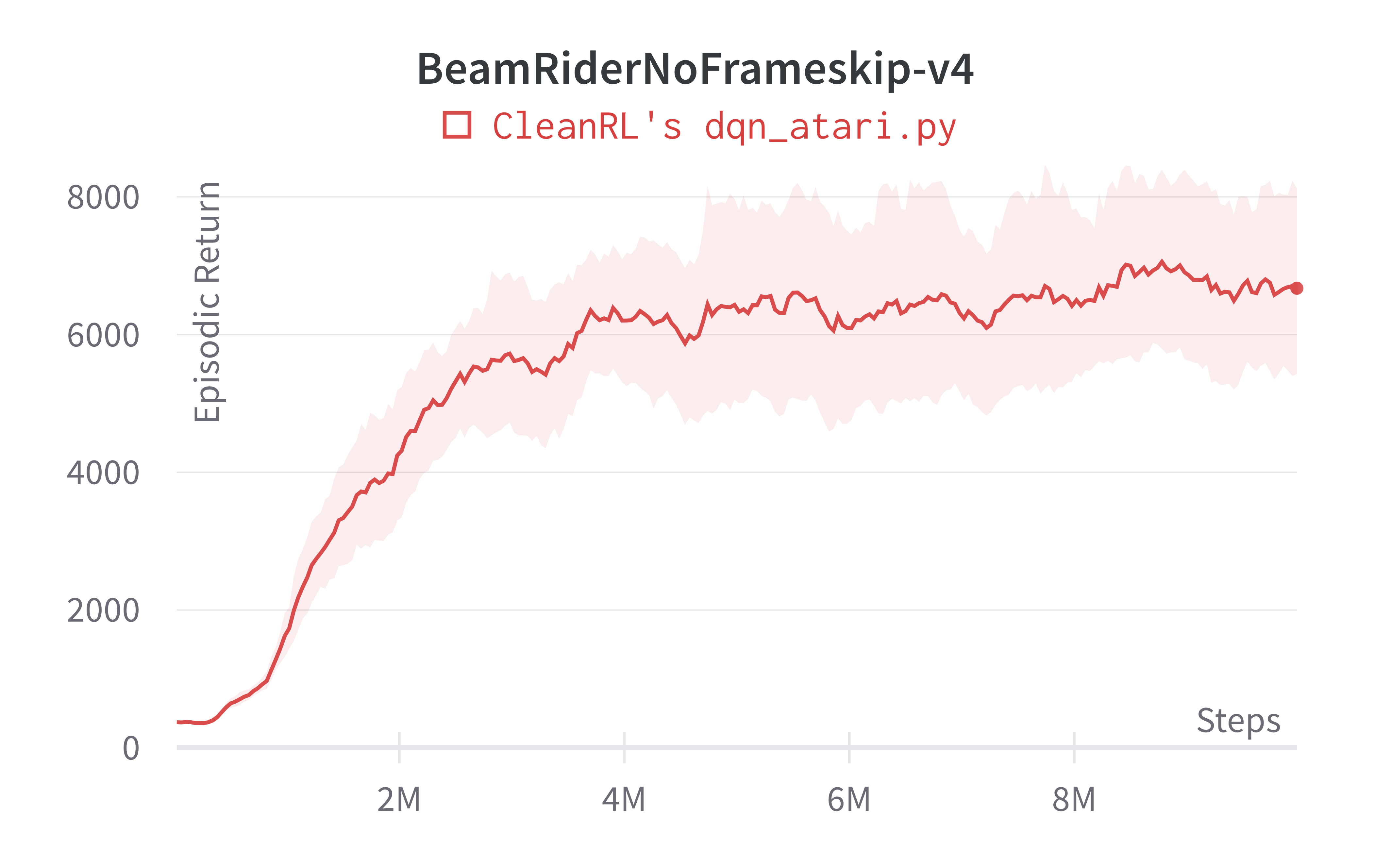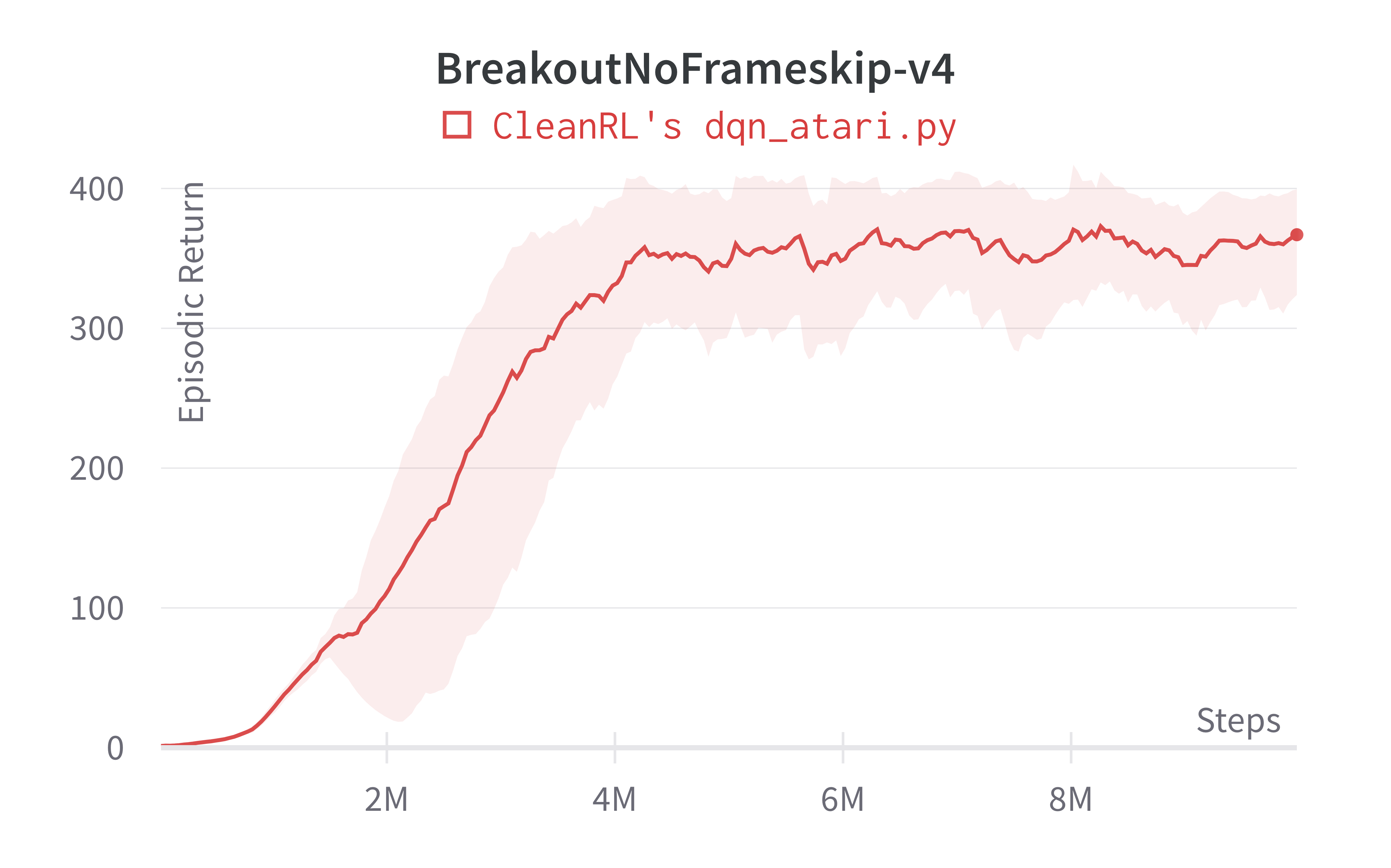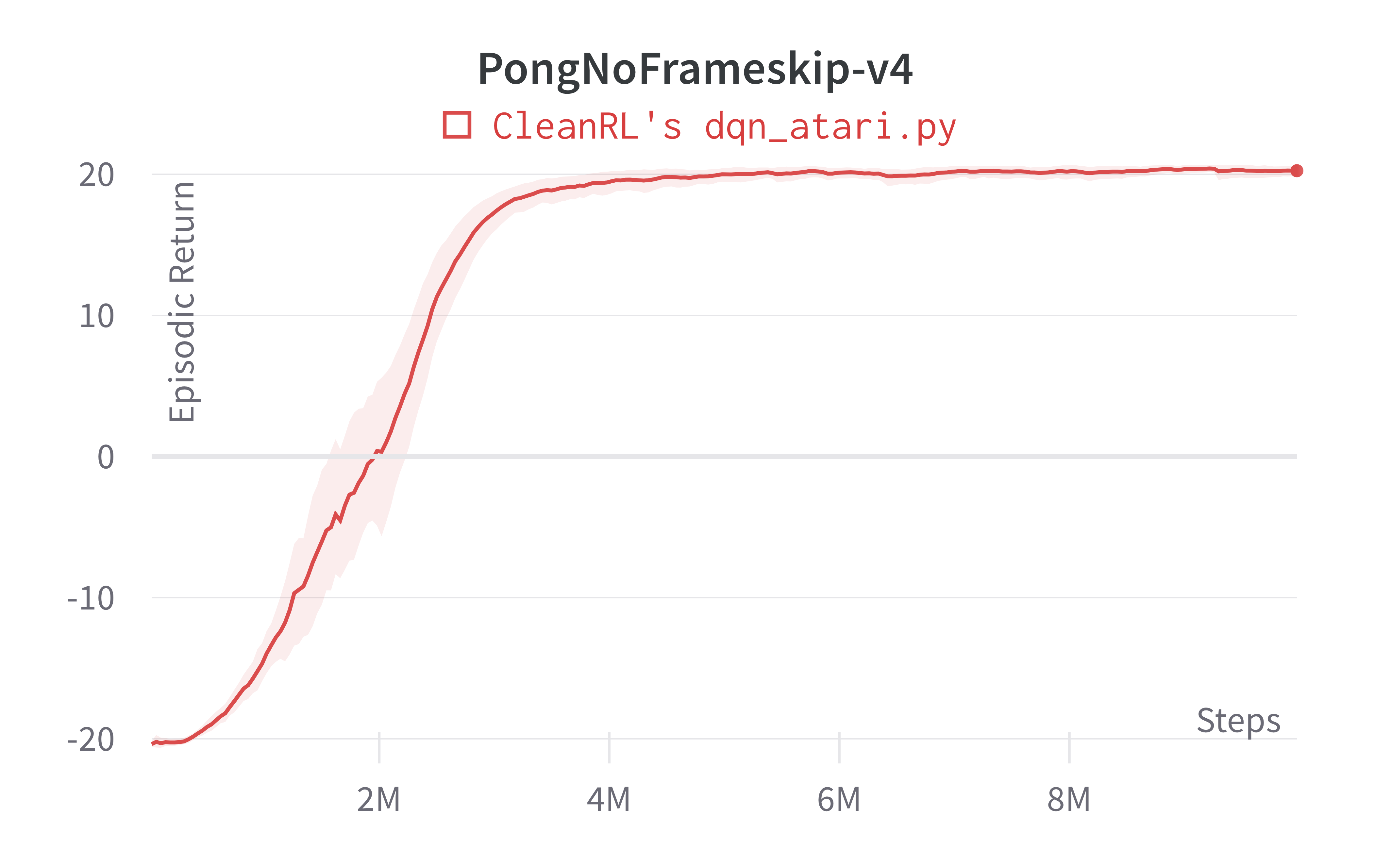Tracked experiments and game play videos:

## dqn.py

The dqn.py has the following features:

• Works with the Box observation space of low-level features
• Works with the Discrete action space
• Works with envs like CartPole-v1

### Usage

poetry run python cleanrl/dqn.py --env-id CartPole-v1

python cleanrl/dqn.py --env-id CartPole-v1


### Explanation of the logged metrics

See related docs for dqn_atari.py.

### Implementation details

The dqn.py shares the same implementation details as dqn_atari.py except the dqn.py runs with different hyperparameters and neural network architecture. Specifically,

1. dqn.py uses a simpler neural network as follows:
self.network = nn.Sequential(
nn.Linear(np.array(env.single_observation_space.shape).prod(), 120),
nn.ReLU(),
nn.Linear(120, 84),
nn.ReLU(),
nn.Linear(84, env.single_action_space.n),
)

2. dqn.py runs with different hyperparameters:

python dqn.py --total-timesteps 500000 \
--learning-rate 2.5e-4 \
--buffer-size 10000 \
--gamma 0.99 \
--target-network-frequency 500 \
--batch-size 128 \
--start-e 1 \
--end-e 0.05 \
--exploration-fraction 0.5 \
--learning-starts 10000 \
--train-frequency 10


### Experiment results

To run benchmark experiments, see benchmark/dqn.sh. Specifically, execute the following command:

Below are the average episodic returns for dqn.py.

Environment dqn.py
CartPole-v1 488.69 ± 16.11
Acrobot-v1 -91.54 ± 7.20
MountainCar-v0 -194.95 ± 8.48

Note that the DQN has no official benchmark on classic control environments, so we did not include a comparison. That said, our dqn.py was able to achieve near perfect scores in CartPole-v1 and Acrobot-v1; further, it can obtain successful runs in the sparse environment MountainCar-v0.

Learning curves: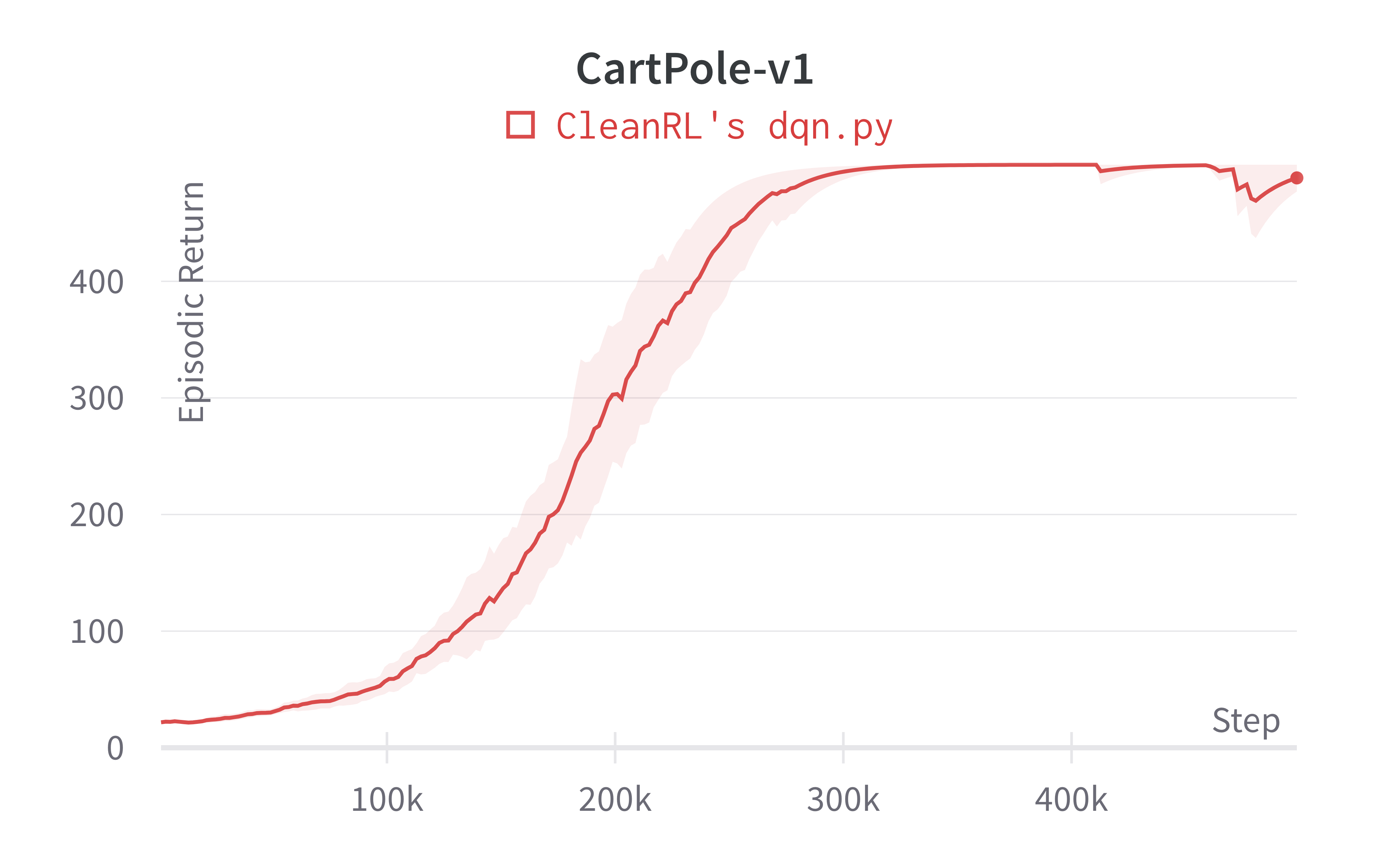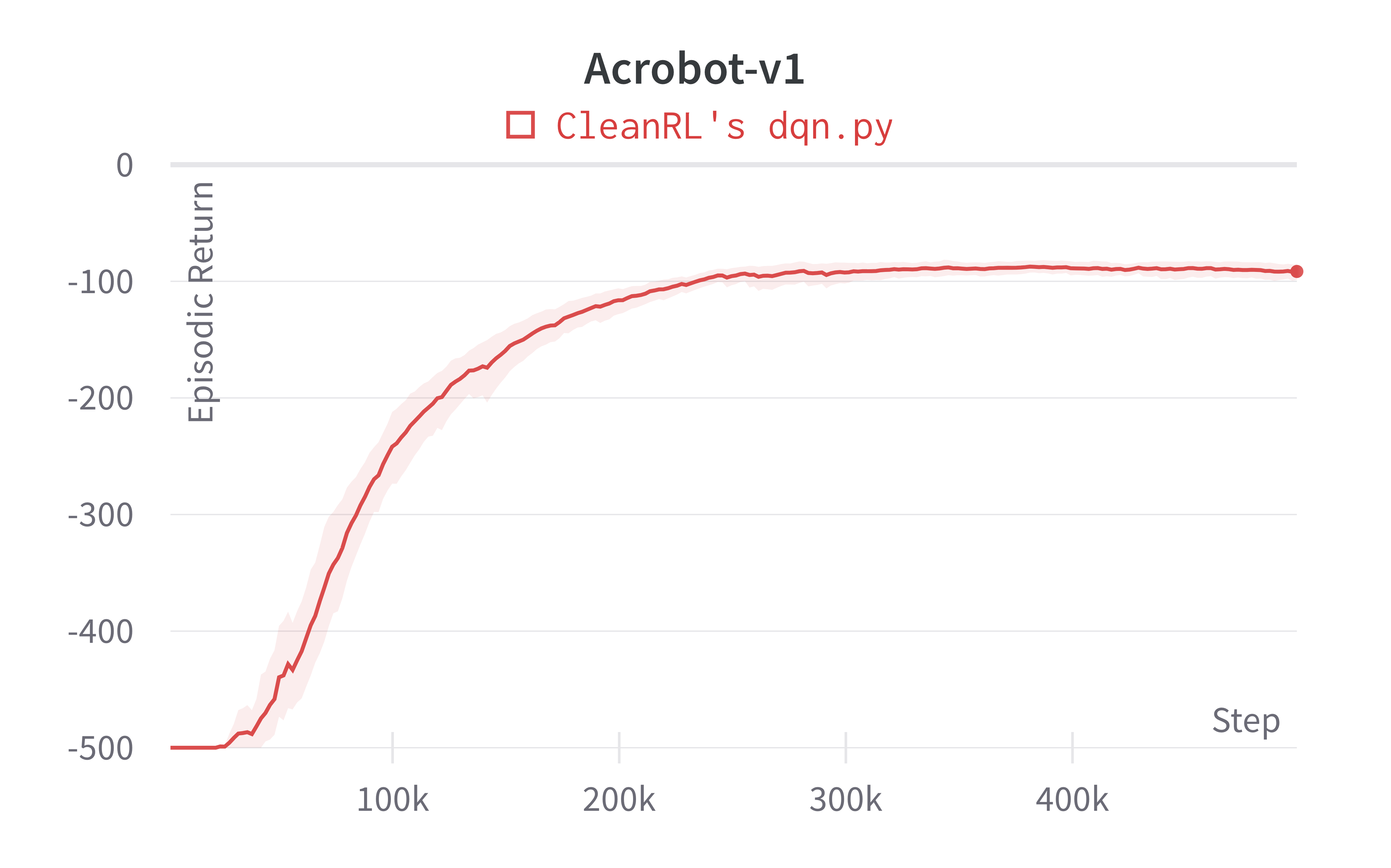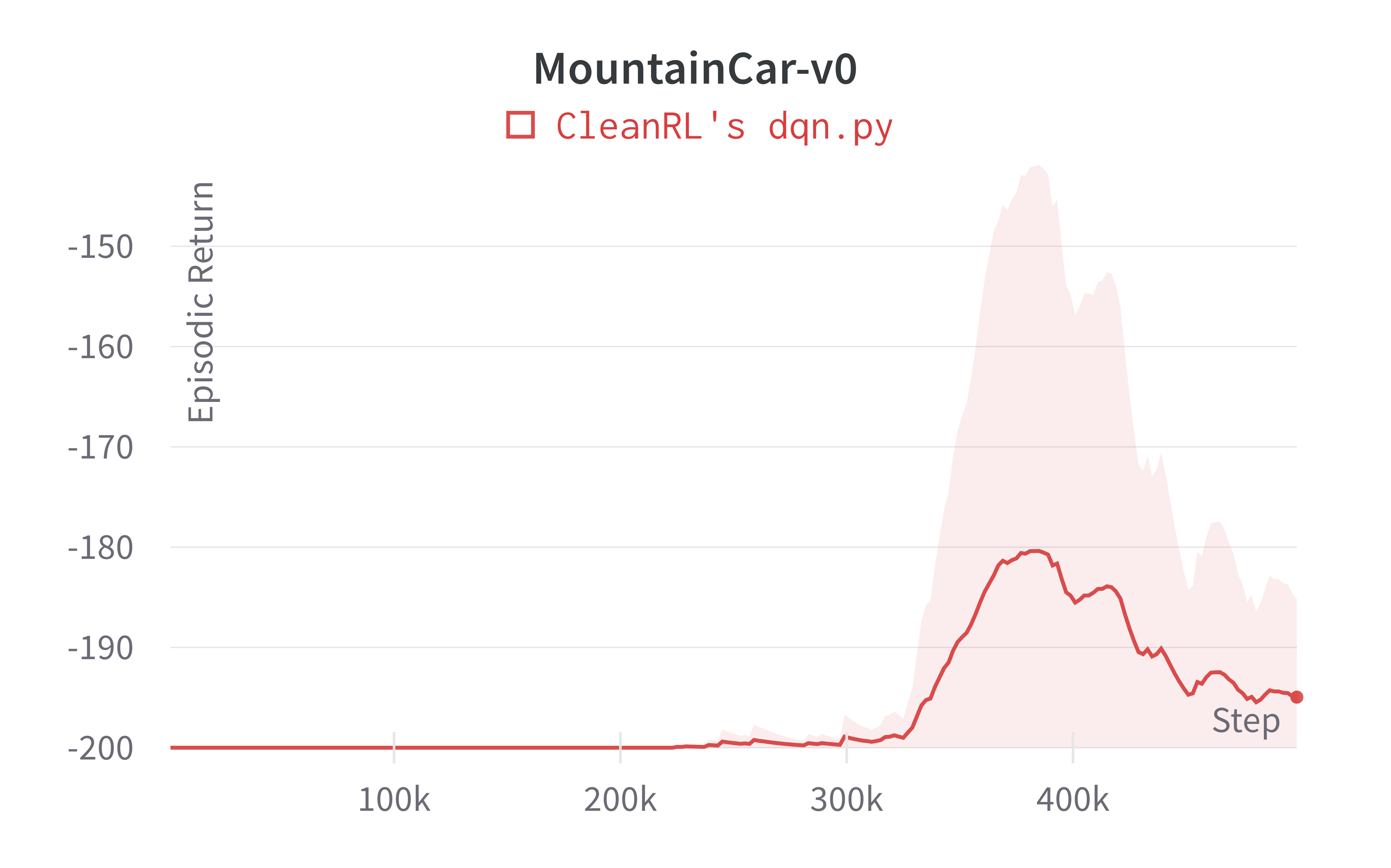Tracked experiments and game play videos:

## dqn_atari_jax.py

The dqn_atari_jax.py has the following features:

• Uses Jax, Flax, and Optax instead of torch. dqn_atari_jax.py is roughly 25%-50% faster than dqn_atari.py
• For playing Atari games. It uses convolutional layers and common atari-based pre-processing techniques.
• Works with the Atari's pixel Box observation space of shape (210, 160, 3)
• Works with the Discrete action space

### Usage

poetry install -E "atari jax"
poetry run python cleanrl/dqn_atari_jax.py --env-id BreakoutNoFrameskip-v4
poetry run python cleanrl/dqn_atari_jax.py --env-id PongNoFrameskip-v4

pip install -r requirements/requirements-atari.txt
pip install -r requirements/requirements-jax.txt
python cleanrl/dqn_atari_jax.py --env-id BreakoutNoFrameskip-v4
python cleanrl/dqn_atari_jax.py --env-id PongNoFrameskip-v4

Warning

Note that JAX does not work in Windows . The official docs recommends using Windows Subsystem for Linux (WSL) to install JAX.

### Explanation of the logged metrics

See related docs for dqn_atari.py.

### Implementation details

See related docs for dqn_atari.py.

### Experiment results

To run benchmark experiments, see benchmark/dqn.sh. Specifically, execute the following command:

Below are the average episodic returns for dqn_atari_jax.py (3 random seeds).

Environment dqn_atari_jax.py 10M steps dqn_atari.py 10M steps (Mnih et al., 2015)1 50M steps (Hessel et al., 2017, Figure 5)3
BreakoutNoFrameskip-v4 377.82 ± 34.91 366.928 ± 39.89 401.2 ± 26.9 ~230 at 10M steps, ~300 at 50M steps
PongNoFrameskip-v4 20.43 ± 0.34 20.25 ± 0.41 18.9 ± 1.3 ~20 10M steps, ~20 at 50M steps
BeamRiderNoFrameskip-v4 5938.13 ± 955.84 6673.24 ± 1434.37 6846 ± 1619 ~6000 10M steps, ~7000 at 50M steps
Info

We observe a speedup of ~25% in 'dqn_atari_jax.py' compared to 'dqn_atari.py'. This could be because the training loop is tightly integrated with the experience collection loop. We run a training loop every 4 environment steps by default. So more time is utilised in collecting experience than training the network. We observe much more speed-ups in algorithms which run a training step for each environment step. E.g., DDPG

Learning curves: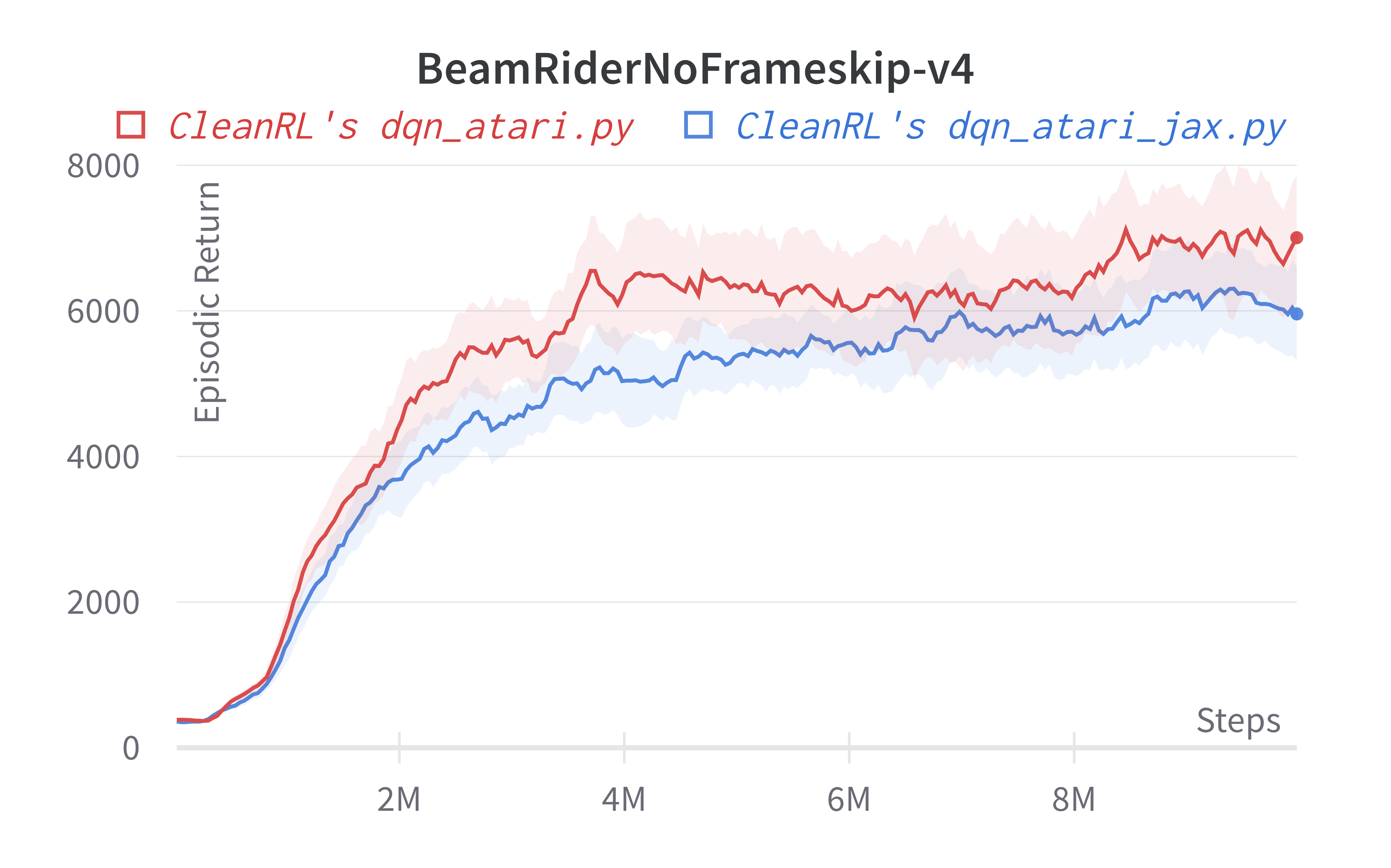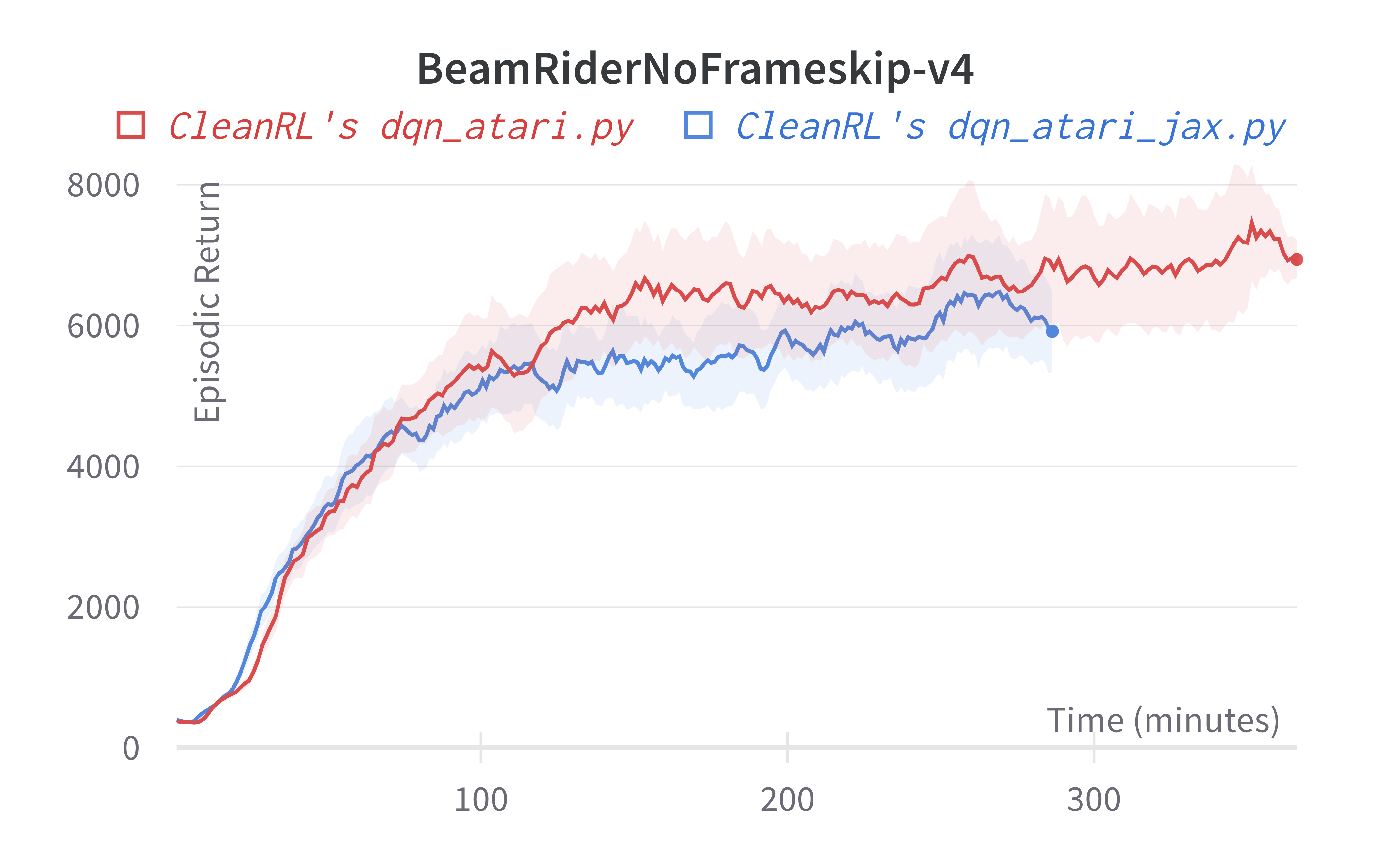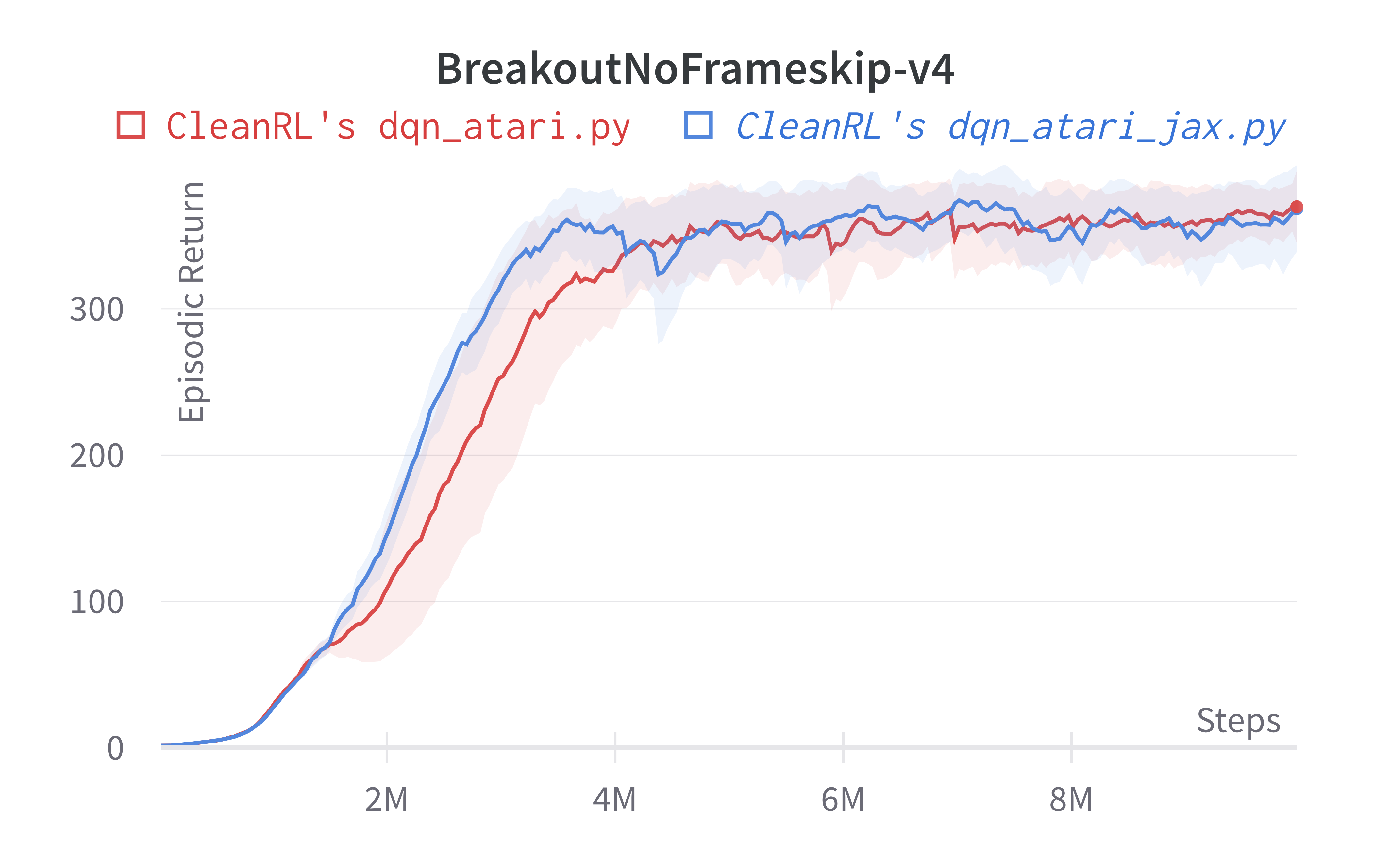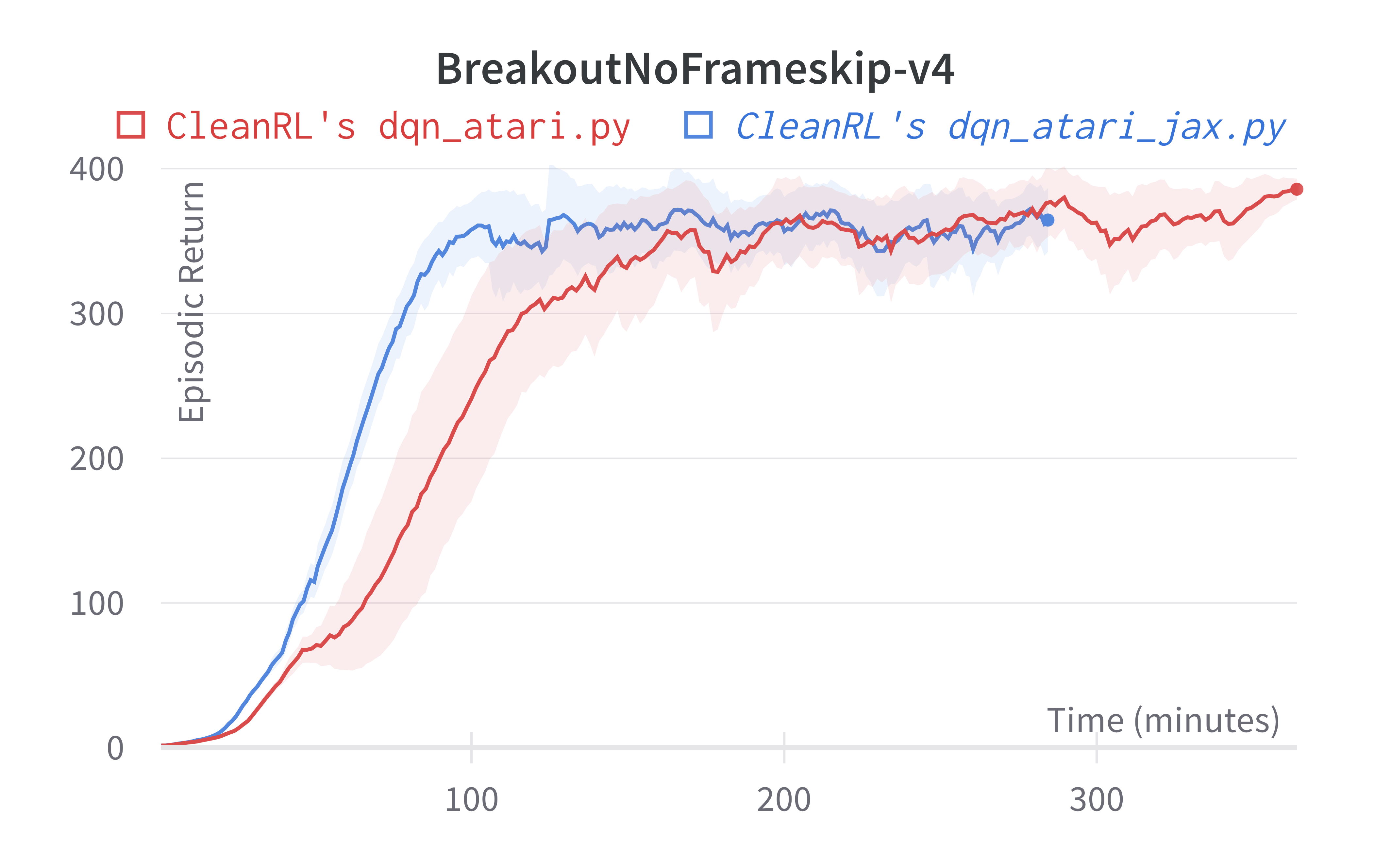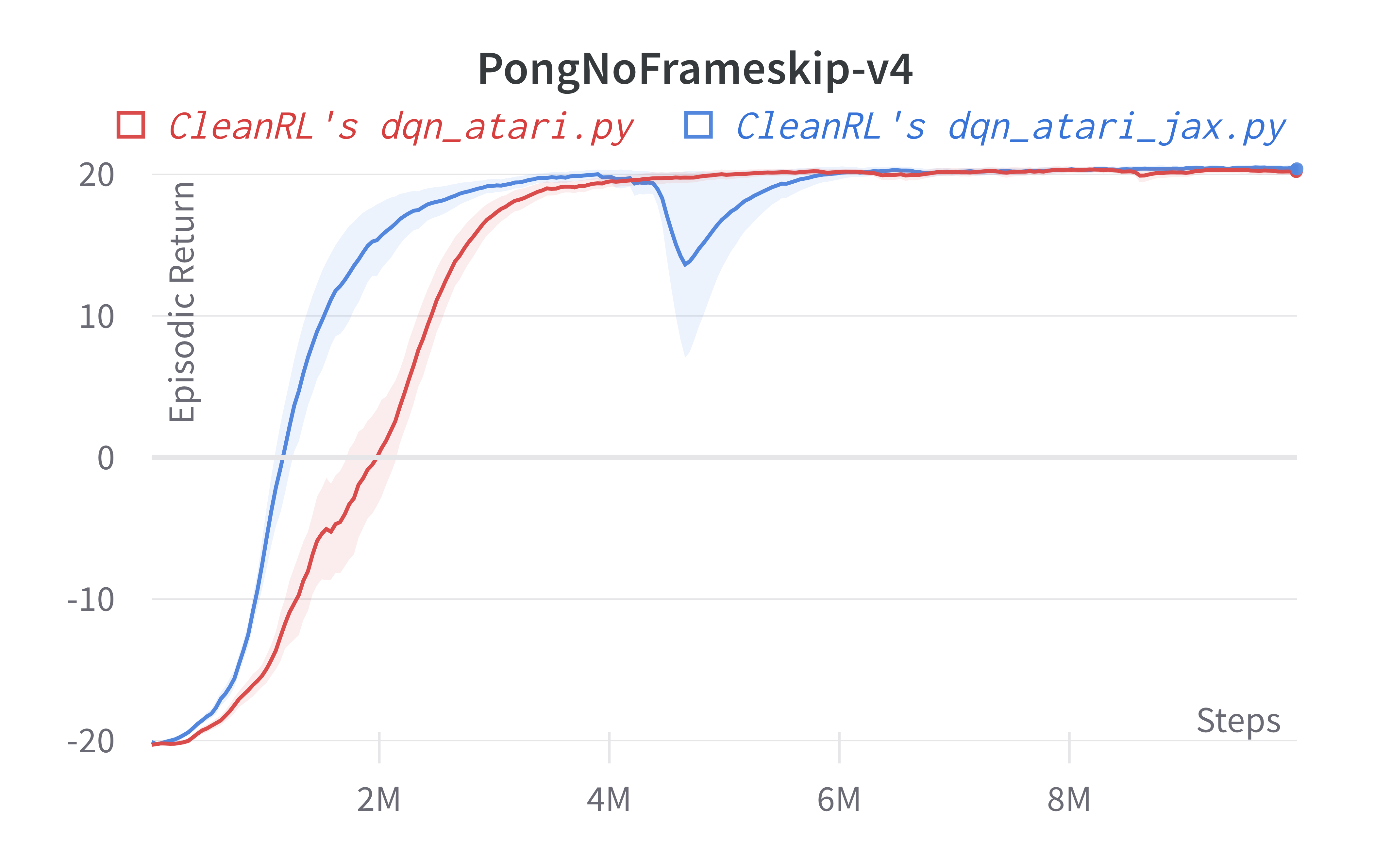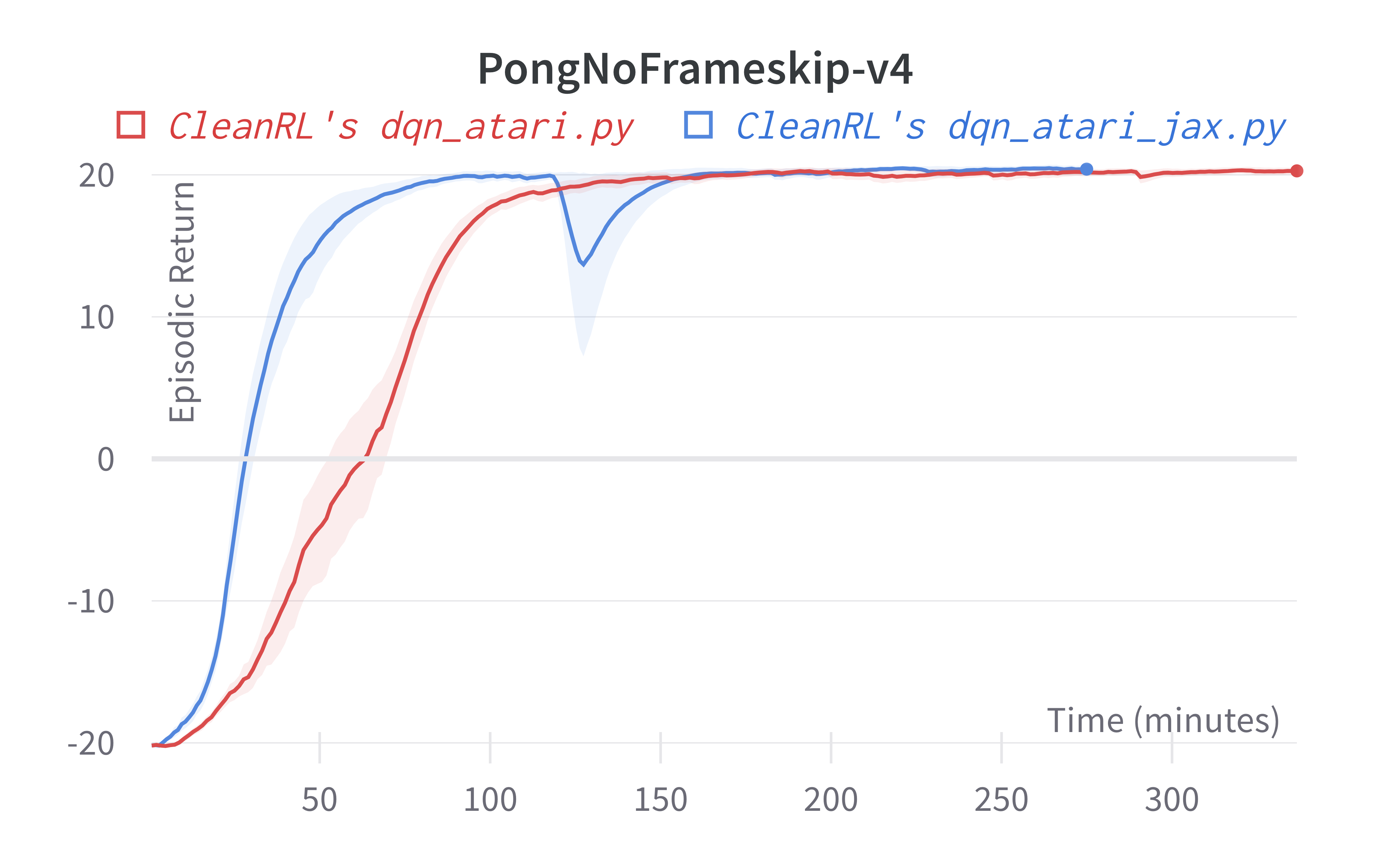Tracked experiments and game play videos:

## dqn_jax.py

• Uses Jax, Flax, and Optax instead of torch. dqn_jax.py is roughly 50% faster than dqn.py
• Works with the Box observation space of low-level features
• Works with the Discrete action space
• Works with envs like CartPole-v1

### Usage

python cleanrl/dqn_jax.py --env-id CartPole-v1

poetry run pip install --upgrade "jax[cuda]==0.3.17" -f https://storage.googleapis.com/jax-releases/jax_cuda_releases.html
poetry run python cleanrl/dqn_jax.py --env-id CartPole-v1

pip install -r requirements/requirements-jax.txt
python cleanrl/dqn_jax.py --env-id CartPole-v1


### Explanation of the logged metrics

See related docs for dqn_atari.py.

### Implementation details

See related docs for dqn.py.

### Experiment results

To run benchmark experiments, see benchmark/dqn.sh. Specifically, execute the following command:

Below are the average episodic returns for dqn_jax.py (3 random seeds).

Environment dqn_jax.py dqn.py
CartPole-v1 498.38 ± 2.29 488.69 ± 16.11
Acrobot-v1 -88.89 ± 1.56 -91.54 ± 7.20
MountainCar-v0 -188.90 ± 11.78 -194.95 ± 8.48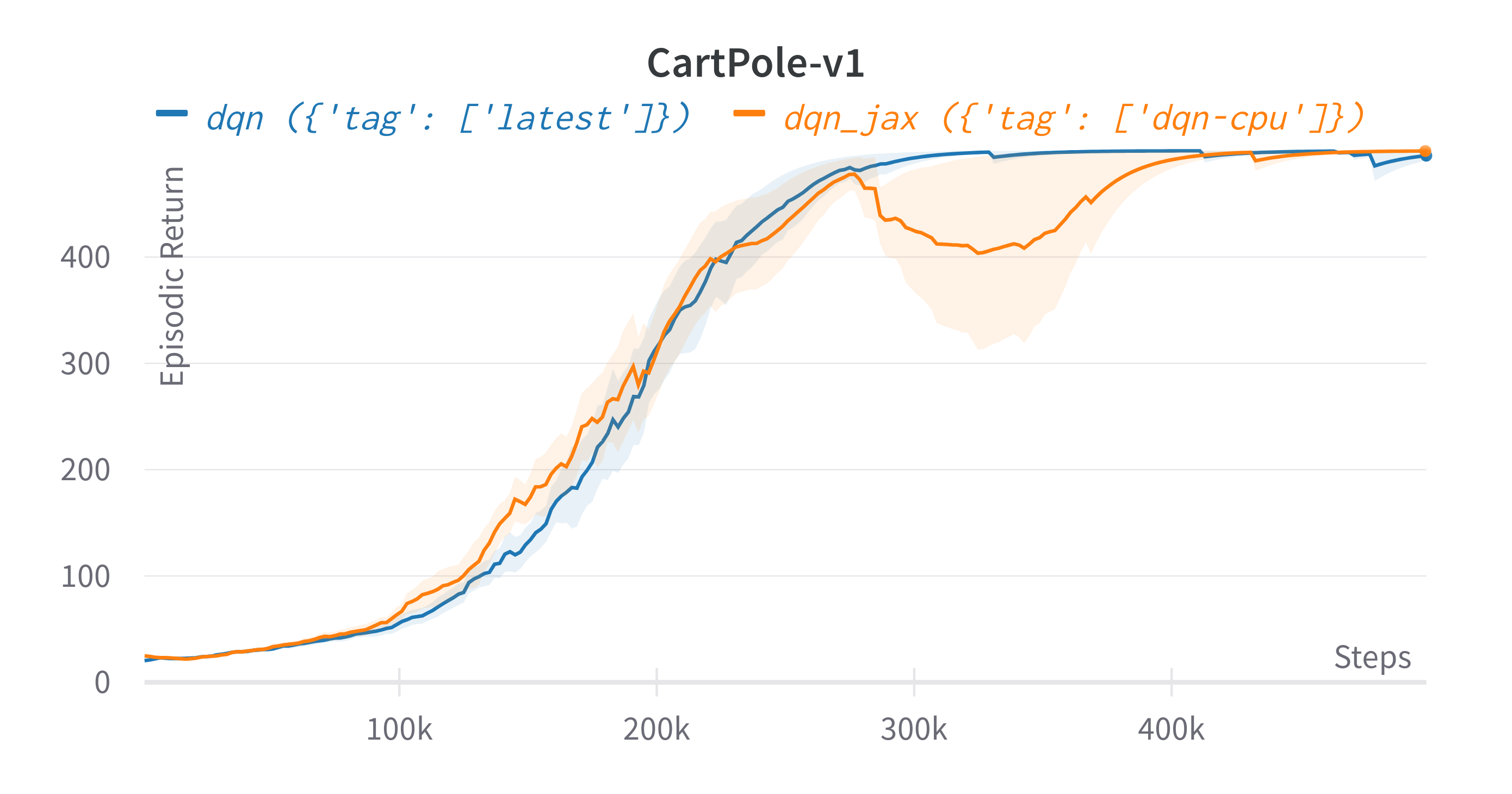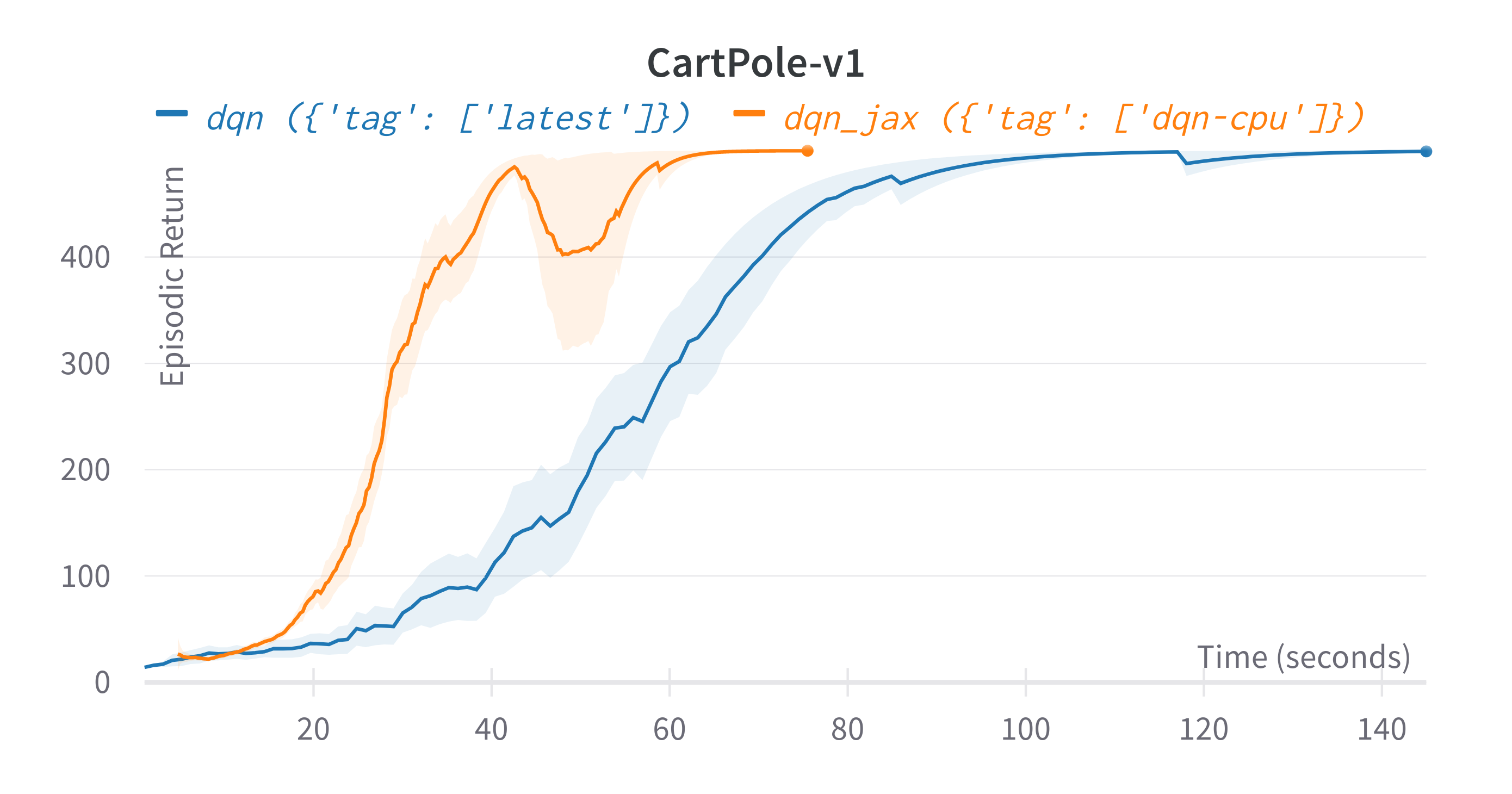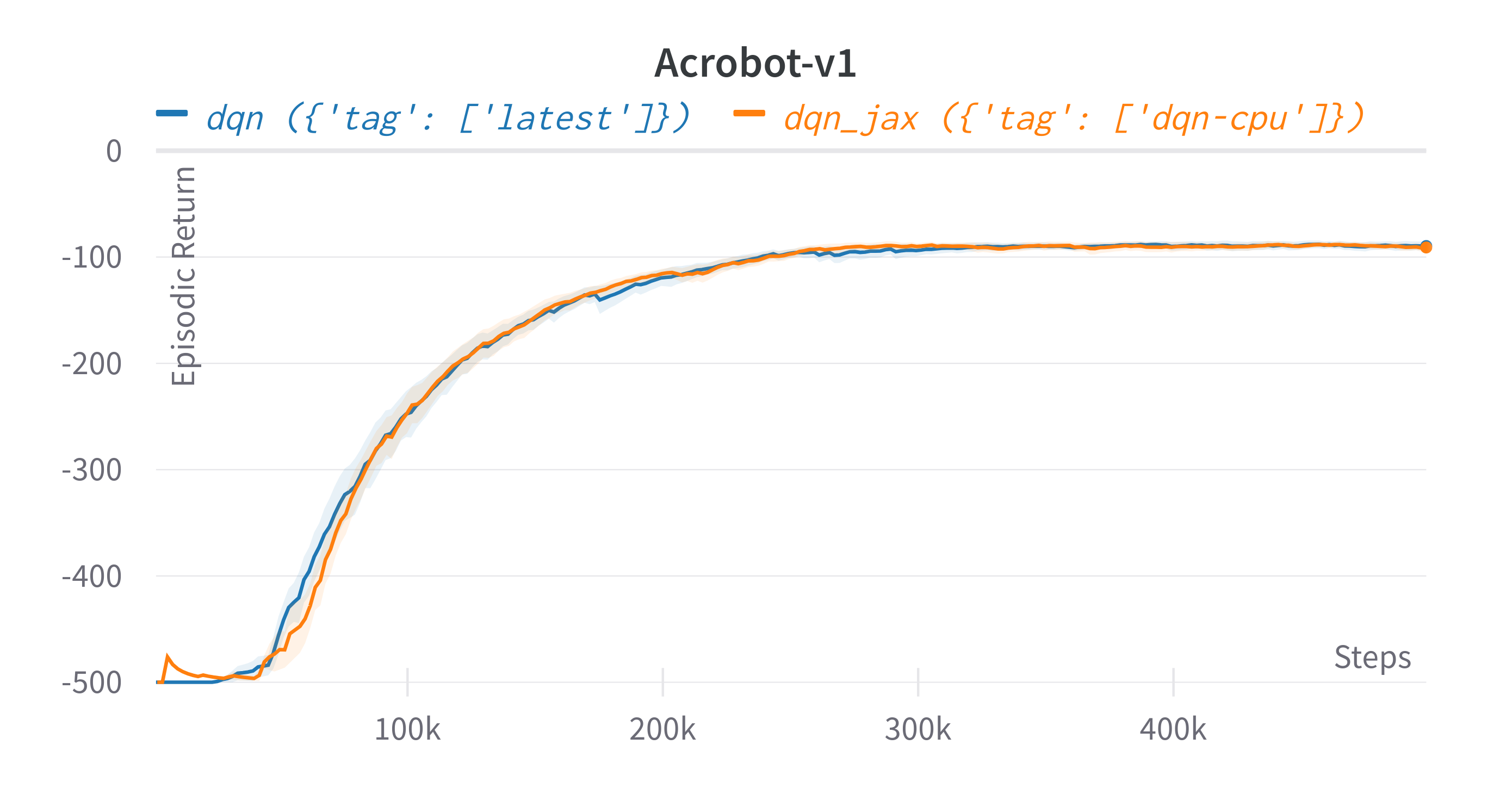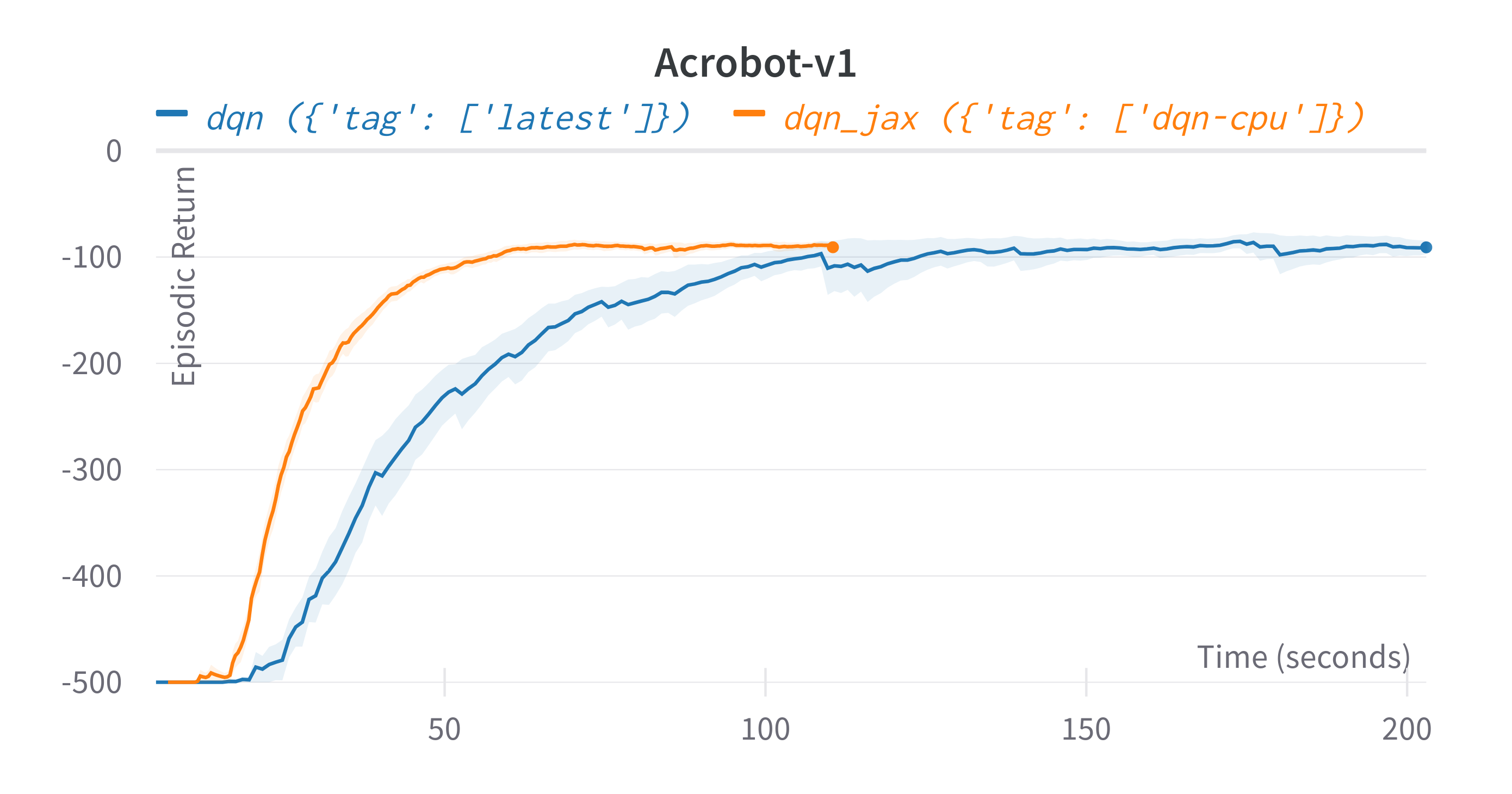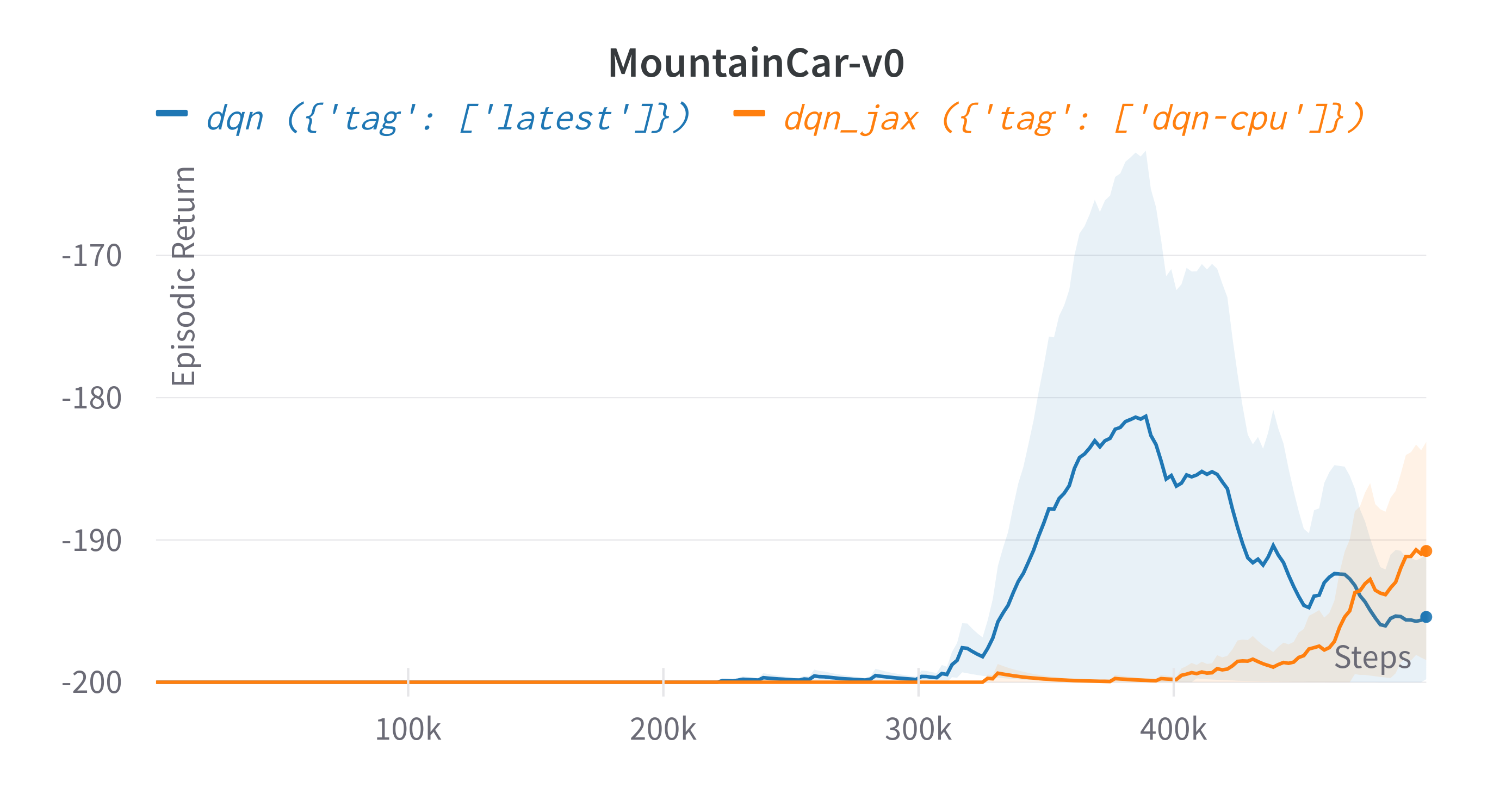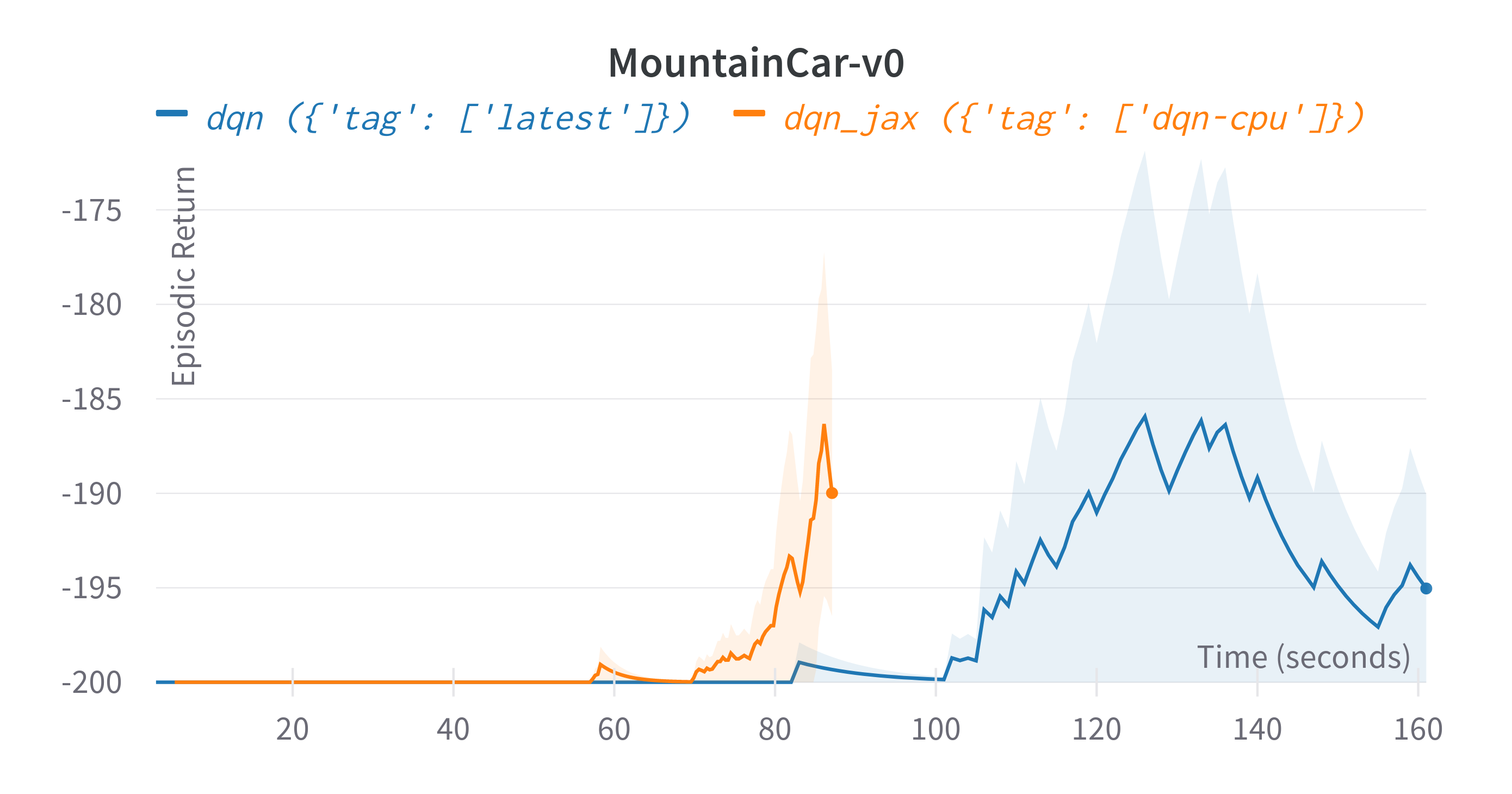Tracked experiments and game play videos:

1. Mnih, V., Kavukcuoglu, K., Silver, D. et al. Human-level control through deep reinforcement learning. Nature 518, 529–533 (2015). https://doi.org/10.1038/nature14236

2. [Proposal] Formal API handling of truncation vs termination. https://github.com/openai/gym/issues/2510

3. Hessel, M., Modayil, J., Hasselt, H.V., Schaul, T., Ostrovski, G., Dabney, W., Horgan, D., Piot, B., Azar, M.G., & Silver, D. (2018). Rainbow: Combining Improvements in Deep Reinforcement Learning. AAAI.